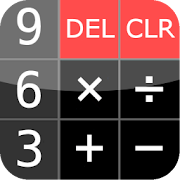# PG Calculator (Standard)Everyone
12
Do you need a simple calculator which allows you to do basic mathematical operations? Or perhaps you are more demanding user and need to calculate trigonometric functions or logarithms ?

Do you prefer to calculate complete algebraic expressions... or maybe you are a fan of Reverse Polish Notation (RPN) ?

Would you like to have a calculator which looks like 'real' on the screen of your smartphone ?

All of this and much more is offered by PG Calculator - the only calculator you will ever need.

PG Calculator is an application that turns your smartphone or tablet into a powerful scientific calculator with nice and simple user interface.

PG Calculator offers two operational modes: algebraic and RPN:
* In algebraic mode you can enter the entire math expressions such as (2+3)*(4-1) or sin(Pi/3)+cos(Pi/6). It then gives you the correct result according to the conventional mathematical operators.
* In RPN (Reverse Polish Notation) mode you first input your numbers and then tell calculator what to do with them. So for example if you want to calculate (3+4)*5 you simply type as follows: 3 ENTER 4 ENTER + 5 ENTER *. The ENTER key places the number on stack and the operator keys do the task on the numbers

Key features:
* Two operational modes: algebraic and RPN;
* Basic mathematical operations such as addition, subtraction, multiplication and division;
* Exponentiation and the calculation of square roots;
* Basic and inverse trigonometric functions;
* The natural logarithms and the decimal;
* Calculator allows you to enter numbers in decimal, binary, octal and hexadecimal;
* Calculator offers the possibility to change the appearance of the user interface with built-in 'skins';
Collapse

## Reviews

Review policy and info
4.6
12 total
5
4
3
2
1

Updated
September 5, 2016
Size
1.4M
Installs
1,000+
Current Version
1.6.23
Requires Android
2.3 and up
Content Rating
Everyone
Permissions
Offered By
Piotr Gridniew
Developer
Main St., Kildare, Co. Kildare, Ireland